### Considering both volatility and permanent loss of capital as risk.

Fundamentals 23: This article argues that volatility and permanent loss of capital are not mutually exclusive views of risks. If you consider them different components you can have a more comprehensive approach to risk mitigation. At the same time, you can use Modern Portfolio Theory or CAPM concepts in your fundamental analysis.

There are 2 schools of thought when it comes to investing risk:
• Those who consider it as volatility.
• Those who consider it as permanent loss of capital (PLofC).

I am a long-term value investor and I follow that latter school of thought. Notwithstanding this, I use the Capital Asset Pricing Model (CAPM) to determine the cost of equity. The CAPM of course uses Beta as the measure of risk which in turn is based on volatility.

I also use Modern Portfolio Theory (MPT) concepts in constructing my stock portfolio. MPT is based on volatility as risk.

There is then an anomaly and a dilemma. If you view risk as a PLofC, why use concepts that are based on volatility? I used to justify this by saying that there is no other simple theory for determining the cost of equity.

I believe that many other value investors are in a similar situation. I today consider it wrong to think that these 2 schools of thought are mutually exclusive.

This post makes a case that both volatility and PLoC address different aspects of investing risk.

This is much better than using some mental gymnastics to rationalize using volatility while following the PLofC school.

## Contents

• Volatility
• Modern Portfolio Theory
• Capital Asset Pricing Model
• An integrated risk management framework
• Conclusion
 This blog is reader-supported. When you buy through links in the post, the blog will earn a small commission. The payment comes from the retailer and not from you. Learn more.

There are 2 components of risk - the chance or probability of an outcome and the impact of the outcome. This is irrespective of which school of thought you follow.

It is obvious that if there is no impact there is nothing to lose. Furthermore, if we are certain that a loss would occur, we would not have invested.

So, when there is investment risk, it is because there is uncertainty as well as a loss if the uncertain outcome occurs. This is an important risk concept for risk mitigation. In other words, to reduce risk, we can address the probability, the impact, or a combination of both.

But first, let us look at why volatility can be considered a risk.

## Volatility

Imagine that you invest in a stock that cost x. The outcome is uncertain so the exit price could be less or even more than x. If it is less than x, there is a loss.

Suppose that we have historical data showing the distribution of prices around some expected mean. Refer to Chart 1.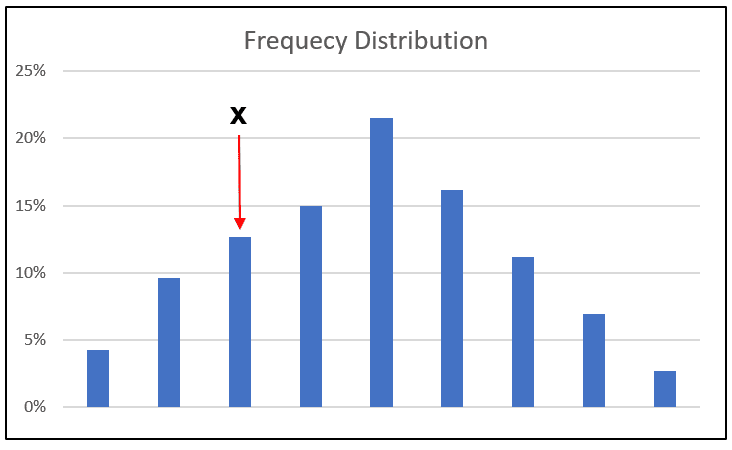Chart 1: Distribution of Prices about the Mean

Statistically, the distribution has a mean. We can measure the distribution of the data around the mean with the standard deviation or variance (square of the standard deviation).

Let us look at this in the context of x.  Based on this distribution we could calculate the frequency of the price being less than x. We can then compare this with all the frequencies and determine the probability of the price being less than x. This can be interpreted as the risk of the stock. You can see that if the standard deviation is bigger, the risk of the price being less than x could be bigger and vice versa.

You can see why the standard deviation or variance can be used to measure risk. A stock with a bigger standard deviation would be considered riskier than one with a smaller standard deviation. Conventionally many use the term “volatility” to describe the variance.

The argument from the PLofC school is that volatility is only crystalized into a permanent loss of capital if you sell when the price is less than x. If you view volatility as temporary and do not sell the stock, you have not suffered any loss. That is why the PLofC school does not believe that volatility is a good measure of risk.

I would argue that there are times when you crystallize the loss even though you know that the price volatility is temporary. For example:
• You sell due to some portfolio rebalancing rule.
• You have made a mistake in estimating the intrinsic value.
• You found a better investment opportunity.

Because of these, it is better to not rule out volatility as a risk even though I follow the PLoC school.

## Modern Portfolio Theory (MPT)

MPT is based on the view that variance represents risk.

When you create a stock portfolio there are two parameters at your disposal.
• You can select the stocks to be included.
• You can also determine the proportion of a stock relative to all the stocks in the portfolio. This is commonly referred to as the weight of the stock in the portfolio. This can be computed based on the cost of the investment or the value of the stocks.

Let us consider the case where you have selected the stocks and are now looking at various portfolio options based on changing the weights. You are looking at the impact on the portfolio return and variance.
• The return of the portfolio is the weighted average return of the individual stocks in the portfolio.
• The variance of the portfolio is not the weighted average variance of the stocks in the portfolio. The portfolio variance is dependent on the variances of the various stocks in the portfolio amended by the covariances. If you have uncorrelated or even lowly correlated stocks, you can have a portfolio variance that is less than the lowest stock variance.

 Case NotesAccording to Investopedia, the standard deviation or variance refers to the spread of a data set around its mean value. The larger the variance, the larger the distance between the numbers in the set and the mean. Conversely, a smaller variance means the numbers in the set are closer to the mean.The covariance refers to the measure of how two random variables will change when they are compared to each other. A positive covariance means both investments' returns tend to move upward or downward in value at the same time. An inverse or negative covariance, on the other hand, means the returns will move away from each other. So, when one rises, the other one falls.Variance covers one variable whereas you need 2 variables to compute the covariance.

You can see the benefits of diversification. The portfolio variance can be reduced with uncorrelated stocks. If you consider variance as a risk then the risk is reduced with the portfolio.

MPT provides a numerical basis for the adage that you should not put all your eggs in one basket.

I have a stock portfolio as part of my risk mitigation plan. While I do not use MPT to construct my stock portfolio, I follow the principle that it should have uncorrelated stocks.

Furthermore, I target about 30 stocks. This is because studies have shown that the benefits of diversification become marginal after this number. These studies generally use volatility as the measure of risk with Chart 2 as a typical one.

You can understand why there would be inconsistencies if I do not consider volatility as a risk.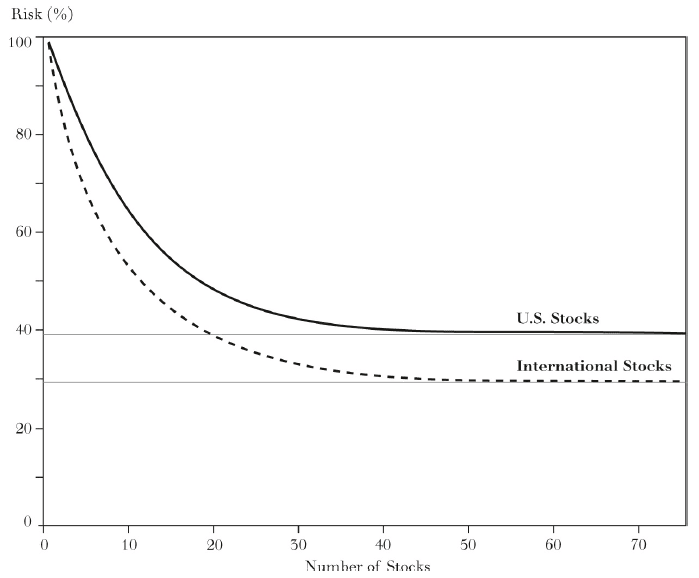Chart 2: Risk vs Number of Stocks in a Portfolio

The PLoC school does not have a quantifiable basis for constructing a stock portfolio.  If you do not consider volatility as risk, can you adopt the MPT approach when constructing a portfolio? You can see the dilemma.

The mental gymnastics I used to get out of this dilemma was as follows:
• While the variance can be considered a measure of risk, covariance is not exactly volatility. But because it can be used to reduce portfolio volatility, people sometimes confuse it with the measure of volatility.
• In other words, although I do not consider volatility as risk, this is ok as MPT focus on covariance rather than variance.

I now think that it is better to consider volatility as another component of risk rather than disregard it completely. Then you do not need some mental gymnastics to rationalize using MPT.

## Capital Asset Pricing Model (CAPM)

I used CAPM to determine the cost of equity. This is then used to determine the WACC which is the discount rate (the expected return) used when estimating the intrinsic value of the firm.

According to Investopedia, CAPM is a finance model that establishes a linear relationship between the required return on investment and risk. And of course, the risk under CAPM refers to volatility.

You can see the anomaly. As a person following the PLoC school, I am relying on a volatility-based theory to determine the cost of equity. I used to justify this by arguing that there was no better theory.

I now believe that it is wrong to treat volatility and PLoC as mutually exclusive. My view now is that they both represent different dimensions of risk.

According to CAPM, the expected return of a stock depends on the stock Beta, the risk-free rate, and the equity risk premium. This is represented by the following equation.

Er = Rf + Beta X (Rm – Rf)

Where:

Er= expected return

Rf = risk-free rate

Rm = market return

Rm – Rf = equity risk premium

The Beta of a stock is a measure of how much risk the stock will add to a market portfolio. If a stock is riskier than the market, it will have a Beta greater than one. If a stock has a Beta of less than one, the formula assumes it will reduce the risk of a portfolio.

The key concept here is that risks are separated into systemic and non-systemic risks.
• Systemic risks refer to the risks inherent to the entire market or market segment. It is also known as “undiversifiable risks,” or “market risks,” as they affect the overall market and not just a particular stock.
• Non-systemic risks refer to risks that are not shared with a wider market or industry. These are risks often specific to an individual company due to its management or business model. Unlike systemic risks, non-systemic risks can be reduced by diversifying one's investments.

Systemic risk is impossible to avoid. It cannot be mitigated by diversification. To account for the systemic risk, you require the stock to deliver a return that is equal to or higher than those determined by the CAPM.

There are 2 takeaways from CAPM when formulating your risk mitigation plan.
• If you hold a concentrated portfolio, you faces more of the non-systemic risks.
• An investor can identify the systemic risk of a particular stock by looking at its Beta. You aim for a higher return when investing in a particular stock relative to that calculated with CAPM.

It is obvious that in addition to seeking a higher expected return, you should also adopt measures that address systemic risks. For example, you could invest in other asset classes.

### Beta

Beta is calculated by dividing the product of the covariance of the stock's returns and the market's returns by the variance of the market's returns over a specified period. It can be represented by the following equation:

Beta = Covariance (Re, Rm) / Variance Rm

Where:

Re =the return on an individual stock

Rm = the return on the market

In statistical terms, Beta represents the slope of the line through a regression of data points. In finance, each of these data points represents an individual stock's returns against those of the market.

You can see from the above equation that Beta relates to volatility.  Beta is a measure of the volatility of a stock compared to the market. Ultimately, you are using Beta to try to gauge how much risk a stock is adding to a portfolio relative to the market risk.
• If a stock has a Beta of 1.0, it indicates that its price activity is strongly correlated with the market. A stock with a Beta of 1.0 has systemic risk. In the Bursa Malaysia context, companies like Hap Seng Consolidated and TH Plantations have levered Beta of around 1.0
• A Beta value that is less than 1.0 means that the stock is theoretically less volatile than the market. Including this stock in a portfolio makes it less risky than the same portfolio without the stock. Examples of Bursa Malaysia companies with a levered Beta significantly less than 1.0 are Suria Capital and Ajinomoto.
• A Beta that is greater than 1.0 indicates that the security's price is theoretically more volatile than the market. Examples of Bursa Malaysia companies with a levered Beta of greater than 1.2 are Notion and Heitech Padu.

 Case NotesWhen looking at Beta, there are 2 points to note:It is usual to differentiate between levered and unlevered Betas.I use the bottom-up Beta approach as per Damodaran.Unlevered Beta (or Asset Beta) measures the market risk of the company without the impact of Debt. Un-levering a Beta removes the financial effects of leverage thus isolating the risk due solely to company assets. In other words, how much did the company's equity contribute to its risk profile?On the other hand, levered Beta (commonly referred to as just Beta or Equity Beta) factors in Debt and Equity. The relationship between the Unlevered Beta and the Levered Beta is given by the following equation: (Source: Investopedia)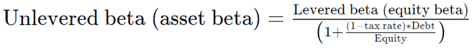According to Professor Damodaran, a bottom-up Beta is estimated by starting with the businesses that a firm is in, estimating the Beta of each of these businesses, and taking a weighted average of these Betas.Bottom-up Betas are better than regression Beta for three reasons:They are more precise. The standard error in a bottom-up Beta estimate is more precise because you are averaging across regression Betas. If a firm has changed its business mix, you can reflect that more easily in a bottom-up Beta because you set the weights on the different businesses. If a firm has changed its Debt to Equity ratio the bottom-up Beta can be easily adjusted to reflect those changes.There are four steps to derive the bottom-up Beta:Step 1: Break your company down into the businesses that it operates in. Step 2: Estimate the Beta of each business. This Beta is called an Asset Beta or an unlevered Beta.Step 3: Take a weighted average of the unlevered Betas of the businesses you are in, weighted by how much value you get from each business.Step 4: Adjust the Beta for your company's financial leverage.If you are a newbie, it could be challenging to consider the various nuances when estimating Beta and computing the WACC. I would recommend that instead of computing the WACC, refer to the many estimates available online. Sites like Seeking Alpha.* present fundamental analysis of companies some of which states the WACC used in the assessment. Tap into them.

## An integrated risk management framework

In my posts “The best way to reduce investment risk” and “Baby steps in assessing Permanent Loss of Capital” I presented a risk management framework comprising several elements.
• The Ishikawa of Fishbone diagram to identify the root causes of a permanent loss of capital.
• A Threat Matrix to assess the likelihood and impact of each of the risks.
• A Risk Mitigation Matrix to ensure that we have measures to mitigate each root cause.

In those articles, I used the framework to analyze risk only as a PLoC. I will now use the same framework but consider both volatility and PLoC.

### Identifying the root causes

In my original article, I identified 4 main causes that can lead to a permanent loss of capital:
• Deterioration of intrinsic value.
• Portfolio construction.
• Wrong initial intrinsic value.
• Stock market changes.

Refer to Chart 3.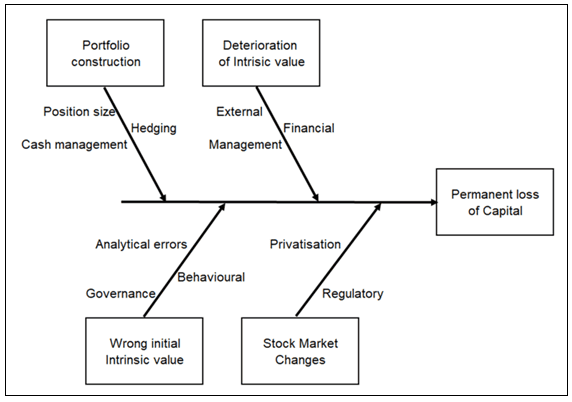Chart 3: Fishbone Diagram with 4 Main Causes of Permanent Loss of Capital

If I now include volatility as another component of risk, I would retain the latter 3 items. However, I would replace the “deterioration in intrinsic value” with 2 other main causes as shown in Chart 4:
• Reduction in intrinsic value due to either systemic or non-systemic risks. You can see that I have sub-causes for the systemic and non-systemic components.
• Temporary volatility that was crystalized to invest in a better opportunity. It can also be for portfolio rebalancing reasons or because of analytical errors.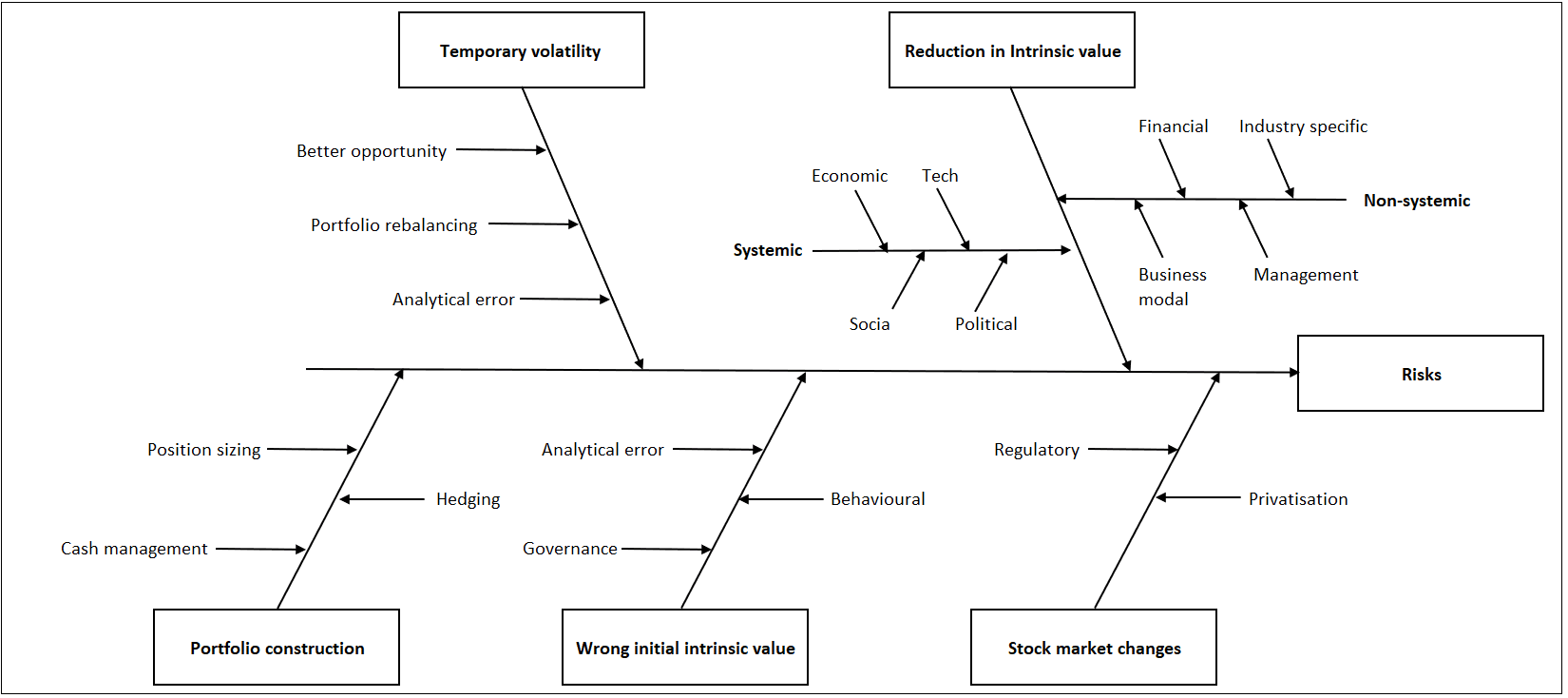Chart 4: Update Fishbone Diagram incorporating Volatility

I have also identified 4 sub-causes each for the systemic and non-systemic components. In other words, to address the systemic and or the non-systemic risks, you address the respective sub-causes.

As for the sub-causes for the Stock market changes, Wrong initial intrinsic value, and Portfolio construction, refer to “Baby steps in assessing Permanent Loss of Capital".

You can see that by considering volatility as risks, there is a more comprehensive picture. The segregation of risks into systemic and non-systemic ones shows that you need to address both if you are not well-diversified. At the same time, you cannot rule out losses due to temporary volatility.

### Threat matrix and risk mitigation measures

Once you have identified the various causes of risks, you can then assess the impact using the Threat matrix. Thereafter you establish the respective risk mitigation measures for each of the causes.

In this article, I will jump straight to the risk mitigation measures. If you want detail on the whole process, refer to “Baby steps in assessing Permanent Loss of Capital".

Chart 5 summarizes the risk mitigation measures for the systemic, non-systemic, and temporary volatility components.  Note the following:
• There is nothing much you can do about the systemic risks. You cannot avoid or reduce them. I have identified 2 measures to live with such risks. The first is to invest in other asset classes such as properties and bonds. The second is to follow the volatility school of aiming for a higher return for taking on the risk. This is achieved by using CAPM to determine the cost of equity.
• Many of the measures listed in Chart 5 are also the ones that I have established under the previous framework.
• There are some cells in Chart 5 where I could not identify a suitable risk mitigation measure. This is not so bad as there are other measures for that particular cause.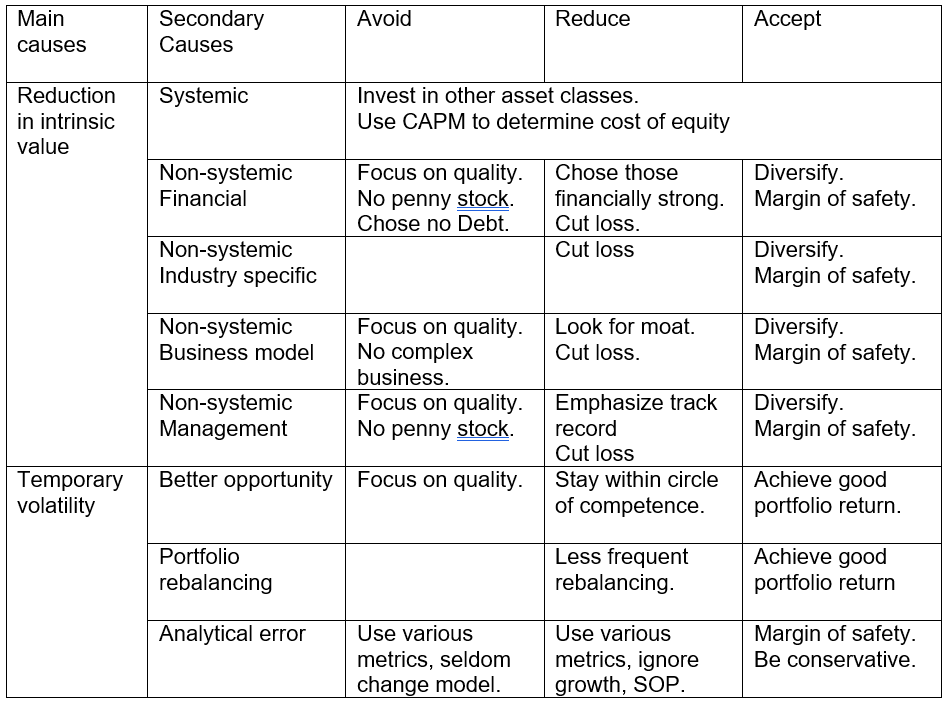Chart 5: My Risk Mitigation Measures

## Conclusion

I am a long-term value investor following the PLoC of thought when it comes to risk. As such I do not view volatility as risks.

The challenge with this is that some of the key concepts I used to analyze and value companies are based on volatility as risks. I have lived with this anomaly for the past 2 decades. This is because the PLoC school does not have a quantitative theory for portfolio construction. At the same time, there is no PloC theory for determining the discount rate.

But there is no need for this anomaly if you view volatility as another risk component. By considering volatility and permanent loss of capital as different components of risks, you can have a more comprehensive picture. At the same time, MPT and CAPM concepts can then be part of your fundamental analysis.

The PLoC school does not have a quantitative measure of risk while the volatility school has managed to do this via the variance. But variance is not a precise measure of risk. But in certain circumstances, an imprecise measure is better than no measure.

I hope that I have made a case that even if you follow the PLoC school, there is a basis for considering volatility.

## End

- - - - - - - - - - - - - - - - - - - - - - - - - - - - - - - - -If the above article was useful, you can find more insights on how to make money in my e-book. The e-book is now available from Amazon, Kobo and Google Play.PS: If you are in Malaysia or Singapore, the e-book can only be download from Kobo and Google Play.

Disclaimer & Disclosure
I am not an investment adviser, security analyst, or stockbroker.  The contents are meant for educational purposes and should not be taken as any recommendation to purchase or dispose of shares in the featured companies.   Investments or strategies mentioned on this website may not be suitable for you and you should have your own independent decision regarding them.

The opinions expressed here are based on information I consider reliable but I do not warrant its completeness or accuracy and should not be relied on as such.

I may have equity interests in some of the companies featured.

This blog is reader-supported. When you buy through links in the post, the blog will earn a small commission. The payment comes from the retailer and not from you.